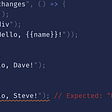# Problem Solving Patterns: Sliding Window

Sliding window is an extremely common problem solving pattern in computer science. It is most frequently used on arrays or strings. The main principle behind it is to create a ‘window’ within your array or string, and then check if the data in that window satisfies the condition of your problem. It can also be used to compare values from different ‘windows’ in your data. For example, lets say you are given an array of integers, and are tasked with finding the three consecutive integers with the greatest sum. You can create a window that spans three indices, and a variable storing the current highest total. Once you have calculated the total of the first window, you move the window forward one index and check if that total is greater. If so, update the total and repeat this process until you have compared all of the data. This problem solving pattern is very helpful when you need to keep track of a subset of your data. If you have spent much time on Leetcode, I’m sure you have seen plenty of problems that a sliding window would work well in, especially ones that involve checking values and characters in arrays and strings.

# Why should I use this pattern?

Much like with the frequency counter pattern(find my article on that here), the sliding window pattern often avoids the need for nested loops. As you will see later in this post when I work through a problem with a sliding window, our sliding window will allow us to iterate through the array or string one time. This pattern can take an O(N²) algorithm and turn it into an O(N) algorithm when applied correctly.

# Solving a problem with a sliding window

The problem I have chosen to solve is Leetcode problem 395: “Maximum Ascending Subarray Sum.” This problem asks us to write a function that takes an array of positive integers as an argument, and returns the maximum possible sum of an ascending subarray. So, the subarray continues as long as the numbers are in ascending order. If the entire array happens to be in ascending order, the return should be sum of all the integers in the array. If the integers are not all in ascending order, the array will be divided into subarrays. For example:

In the image above, the first three integers are in ascending order, but the fourth one is less than the one before. All the integers after 2 increase from there, forming our second subarray. In this case, the second subarray has a sum of 59, which is greater than the first subarray and will be the number that our function should return in this case.

## Setting up our windows

Our window is essentially going to be two pointers. I decided to start with one on the first index in the array, and another on the one following it. I named them ‘i’ and ‘j’, and they will start at 0 and 1, respectively. ‘J’ will keep increasing as long as the integer at the next index is greater than the one at index ‘j’. If the next integer is not greater than the current one, that means it is time to “close” the window. What I mean by this is that ‘i’ will be moved up to the same index as ‘j’, and the process will begin again.

## Keeping track of sums

In order for the approach that I just outlined to work, our function also needs to keep track of different subarrays sums and which one is the greatest. I did this by creating one variable that is the highest sum so far(named ‘max’), and another that is the sum of the current subarray being iterated over(named ‘currentTotal’). I chose to set both of them equal to the first integer of the array, because of the way that I wrote the loop, which is in the picture below.

I decided to set it up so that the current total would only increase if the integer at index ‘j’ was greater than the one at index ‘i’. If yes, the current total should increase by the integer at index ‘j’, and the current total needs to be compared to the max. If the max is less than the current total, it is set to the current total. If the integer at index ‘i’ is the greater of the two, the window needs to be closed, and the current total needs to be reset to the first index of the new window. After the entire array has been iterated over, the max is returned.

# How did I do?

Not bad! My code is faster than 92% of Javascript solutions on Leetcode, and uses less memory than 54%.

--

--

--

## More from Liam Hanafee-Areces

Love podcasts or audiobooks? Learn on the go with our new app.

## Maintain health including.## Let’s Build a Thing! (Part Three)## Building a reusable Component System with React.js and styled-components## Run NestJS Application in Serverless Framework on AWS## 5 Front-End Predictions and Trends for 2020## Practical way to organize your SASS files## LeetCode Problem : Sign of the Product of an Array## Two Sorting Algorithms You Must Know About — Quick Sort and Merge Sort## Let your code tell a story## Top 5 Technical Interview Tips